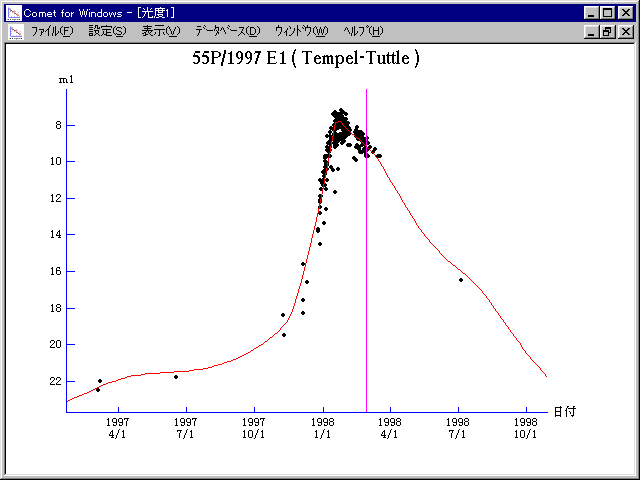# \$B%F%s%Z%k(B-\$B%?%C%H%kWB@1(B

55P/Tempel-Tuttle (1998)###\$B%W%m%U%#!<%k(B

 \$BId9f(B 55P/1997 E1 \$B8!=PF|(B 1997\$BG/(B 3\$B7n(B 4\$BF|(B \$B8!=P8wEY(B 22.5\$BEy(B \$B8!=P K. J. Meech, O. R. Hainaut and J. Bauer (Mauna Kea)

###\$B50F;MWAG(B

```   The following improved orbital elements by Kenji Muraoka,
are from 348 observations 1866 to 1998, including 9 planets,
and 5 minor planets perturbations and non-gravitational effect
of style II.   The mean residual is +/- 1.08 arc seconds.

Epoch  =  1998 Mar.  8.0  TT       JDT = 2450880.5
T  =  1998 Feb. 28.09752       +/- 0.00011 (m.e.) TT
Peri. =  172.49873                +/- 0.00017
Node  =  235.25865                +/- 0.00010   (2000.0)
Incl. =  162.48615                +/- 0.00002
q  =    0.9765761              +/- 0.0000003 AU
e  =    0.9055183              +/- 0.0000000
a  =   10.3361455              +/- 0.0000003 AU
n  =    0.02965966             +/- 0.00000000
P  =   33.231                  +/- 0.0000015  years
A1  =   -0.401                  +/- 0.007
A2  =   +0.00880                +/- 0.00000
```

###\$B@1?^(B1997\$BG/(B 9\$B7n(B29\$BF|!A(B1998\$BG/(B 1\$B7n(B27\$BF|(B1998\$BG/(B 1\$B7n(B27\$BF|!A(B1998\$BG/(B 8\$B7n(B25\$BF|(B

###\$B8wEYJQ2=(B

```        m1 = 15.0 + 5 log\$B&\$(B +  7.0 log r  [    ,-100]  (              \$B!A(B1997\$BG/(B11\$B7n(B20\$BF|(B)
m1 =  7.5 + 5 log\$B&\$(B + 35.0 log r  [-100, -40]  (1997\$BG/(B11\$B7n(B20\$BF|!A(B1998\$BG/(B 1\$B7n(B19\$BF|(B)
m1 =  8.5 + 5 log\$B&\$(B + 20.0 log r  [ -40,    ]  (1998\$BG/(B 1\$B7n(B19\$BF|!A(B               )
```##### \$B50F;MWAG\$OB<2,7r<#;a\$N7W;;\$K\$h\$k\$b\$N\$G\$9!#(B \$B@1?^\$O(B StellaNavigator Ver.2.0 for Windows (\$B%"%9%H%m%"!<%D(B \$BJTCx(B / \$B%"%9%-!<=PHG6I4)(B) \$B\$G:n@.\$7\$?\$b\$N\$G\$9!#(B \$B8wEY%0%i%U\$O(BComet for Windows\$B\$G:n@.\$7\$?\$b\$N\$G\$9!#(B